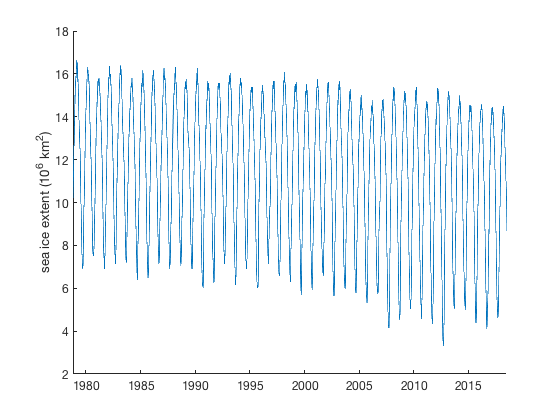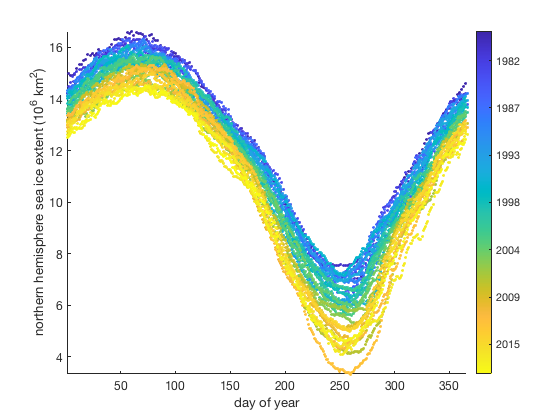# doy documentation

The doy function returns the Julian day of year.

## Syntax

```n = doy(t)
n = doy(t,'decimalyear')
n = doy(t,'remdecimalyear')```

## Description

n = doy(t) gives the day of year (from 1 to 366.999) corresponding to the date(s) given by t. Input dates can be datenum, datetime, or string format.

n = doy(t,'decimalyear') gives the year in decimal form of input date t. It accounts for leap years, so the decimal value for a given date will depend on whether it's a leap year. For example, July 4th of 2016 (a leap year) is 2016.5082 whereas July 4th of 2017 (not a leap year) is 2017.5068.

n = doy(t,'remdecimalyear') returns only the remainder of the decimal year, and is always in the range 0 to 1.

## Example 1: datestr format

What's the Julian day of the year for Valentine's Day?

```doy('february 14')
```
```ans =
45.00
```

45. That's because there are 31 days in January, and Valentine's Day is on the 14th of February.

## Example 2: datenum format

As I write this example file, it is

```>>now
ans =
737427.95```

which means it has been 737427 days since January first of the year zero. So what day of the year is it right now?

```doy(737427.95)
```
```ans =
2.95
```

As you can see, it is late at night on January 2nd. Only 5% of the day remains.

## Example 3: datetime format

Consider this sea ice extent data:

```load seaice_extent.mat

whos extent_N t % displays sizes of these variables
```
```  Name              Size             Bytes  Class       Attributes

extent_N      12854x1             102832  double
t             12854x1             205665  datetime

```

The variable t is in datetime format, and it contains one date just about every from 1978 to 2018. Here's the time series:

```plot(t,extent_N)
ylabel 'sea ice extent (10^6 km^2)'
box off % removes the ugly frame
```Plot the data as a function of Julian day like this

```jday = doy(t);

scatter(jday,extent_N,10,datenum(t),'filled')
cb = cbdate('yyyy');     % formats the colorbar as dates
set(cb,'ydir','reverse') % flips the colorbar axis
axis tight
ylabel 'northern hemisphere sea ice extent (10^6 km^2)'
xlabel 'day of year'
```## Author Info

This function is part of the Climate Data Toolbox for Matlab. The function and supporting documentation were written by Chad A. Greene of the University of Texas at Austin.